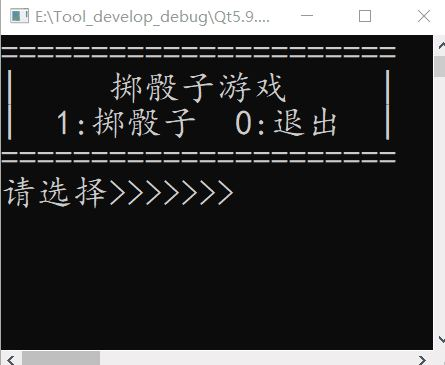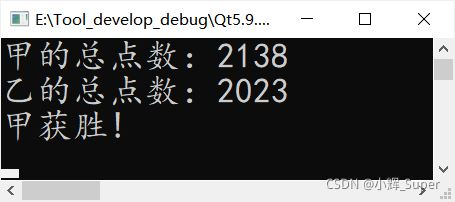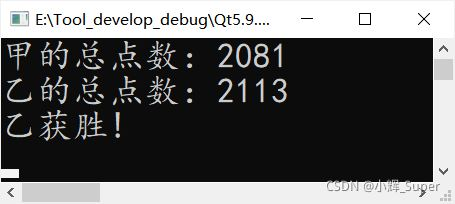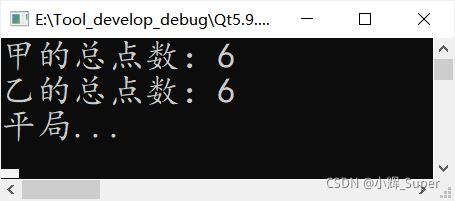# C语言模拟掷骰子游戏

## 实现掷骰子小游戏

### 实现原理

——百度百科

`srand`函数是随机数发生器的初始化函数。原型：`void srand(unsigned int seed)`;
`srand``rand()`配合使用产生伪随机数序列。

`rand()`

srand()：

——百度百科

`time() `是指返回自 Unix 纪元（January 1 1970 00:00:00 GMT）起的当前时间的秒数的函数，主要用来获取当前的系统时间，返回的结果是一个`time_t`类型。
`time_t time(time_t *t);`

——百度百科

`time_t`的原型就是`long int`，这个函数的实现原理我们也没必要去深究，我们只要清楚它能返回秒数就足够了。

```srand((unsigned)time(NULL)); //用系统秒数初始化随机数种子
ret = rand();                //产生随机数，赋值给ret变量```

`ret = rand() % 6 + 1;       //ret即为随机点数`

### 源码介绍

• 菜单打印：打印功能选择，显示上次投骰子的结果
• 操作选择：选择掷骰子或退出退出程序
```#include <stdio.h>
#include <stdlib.h>     //srand()/rand()
#include <time.h>       //time()

int main()
{
char choice = '\0'; //菜单选择
int ret = 0;
//用系统秒数初始化随机数种子
srand((unsigned)time(NULL));
while(1)
{
/* 1.菜单打印 */
system("cls");  //清屏--Windows系统
printf("======================\n");
printf("|     掷骰子游戏     |\n");
printf("|  1:掷骰子  0:退出  |\n");
if(ret)
printf("|     上轮点数:%d     |\n", ret);
printf("======================\n");

/* 2.操作选择 */
printf("请选择>>>>>>>\n");
scanf(" %c", &choice);    //输入选择
//" %c" 可以吃前面的空格和回车
while(getchar() != '\n'); //吃掉除choice外其它字符
ret = 0;                  //初始化点数
switch(choice)
{
case '0':
printf("Goodbye!\n");
return 0;   //退出
//掷骰子(产生1 ~ 6 随机数)
case '1':
ret = rand() % 6 + 1;
break;
default: printf("无效选择!\n");
}
}
return 0;
}
```

### 效果展示## 今日练习

### 代码实现

```#include <stdio.h>
#include <stdlib.h>
#include <time.h>

int main()
{
int sum_1 = 0, sum_2 = 0;
int i = 0, j = 0;
//用系统秒数初始化随机数种子
srand((unsigned)time(NULL));
for(i = 0; i < 100; i++)
{
for(j = 0; j < 6; j++)
{
//获取骰子点数
sum_1 += rand() % 6 + 1;
sum_2 += rand() % 6 + 1;
}
}
printf("甲的总点数：%d\n", sum_1);
printf("乙的总点数：%d\n", sum_2);
if(sum_1 > sum_2)
printf("甲获胜!\n");
else if(sum_1 == sum_2)
printf("平局...\n");
else
printf("乙获胜!\n");
return 0;
}
```

### 运行结果0
0

0 条回复（回复会通过微信通知作者）

（微信扫码即可登录，无需注册）